Question

1. When 2.50 g of copper metal reacts with molecular oxygen, the copper oxide product of the reaction has a mass of...

1. When 2.50 g of copper metal reacts with molecular oxygen, the copper oxide product of the reaction has a mass of 2.81 g. What is the empirical formula of the copper oxide product

2. When 10.8 g of silver was reacted with sulfur. 12.4 grams of product was produced there was only one product). What is the empirical formula of the product?

3. Lysine is a compound composed of carbon, hydrogen, nitrogen and oxygen. When 1.50 g of lysine is burned, 2.72 g of carbon dioxide, 1.29 g of water and 0.287 g of nitrogen gas are produced. What is the empirical formula of lysine? If the molar mass of lysine is 146.19 g/mol, what is the molecular formula?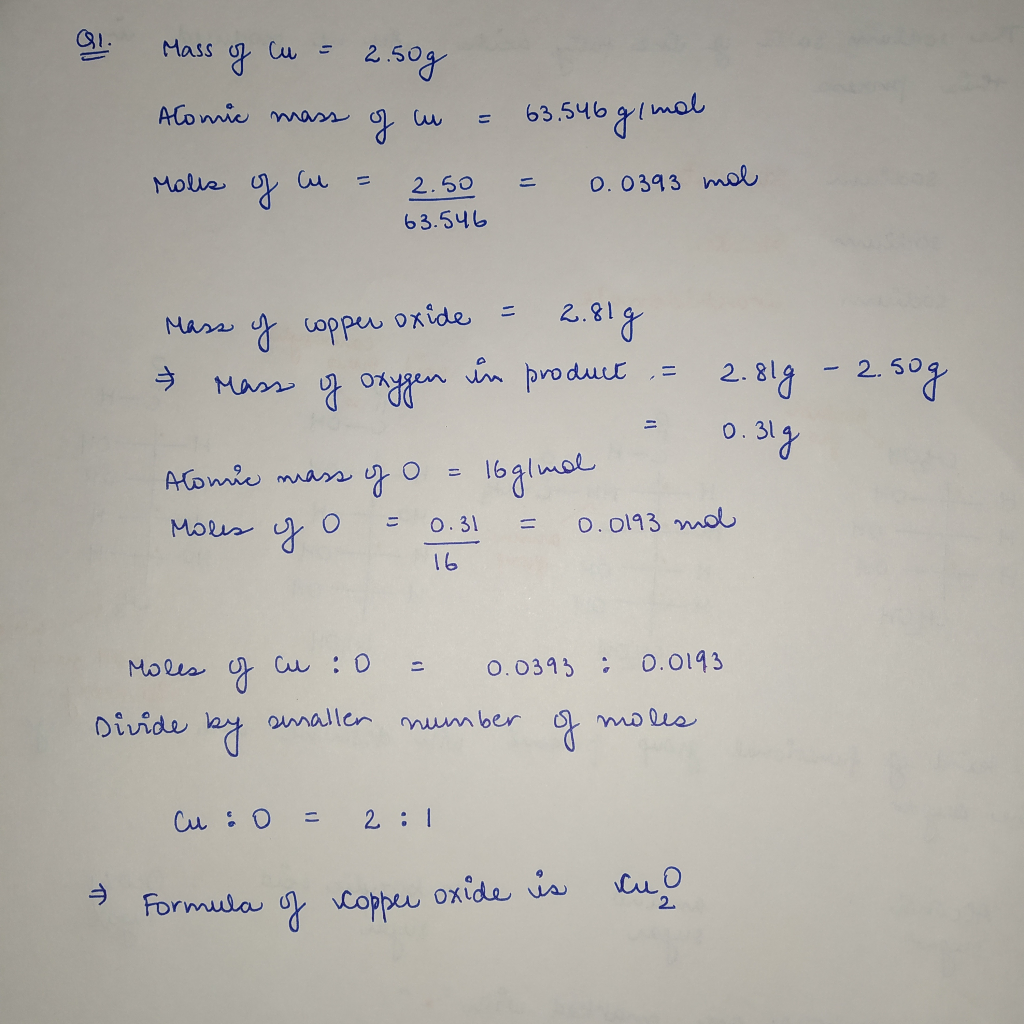Earn Coins

Coins can be redeemed for fabulous gifts.

Similar Homework Help Questions
• 1. A 0.750-g sample of tin metal reacts with 0.201 g of oxygen gas to form tin oxide.

1. A 0.750-g sample of tin metal reacts with 0.201 g of oxygen gas to form tin oxide. Calculate the empirical formula of the tin oxide. 2. A 0.565-g sample of cobalt metal reacts with excess sulfur to give 1.027 g of cobalt sulfide. Calculate the empirical formula of the product. 3. A 1.164-g sample of iron filings reacts with chlorine gas to give 3.384 g of iron chloride. Calculate the empirical formula of the product? 4. A 0.626-8 sample of copper oxide...

• 5. A 0.565-8 sample of cobalt metal reacted with excess sulfur powder to give 1.027 g of cobalt sulfide

5. A 0.565-8 sample of cobalt metal reacted with excess sulfur powder to give 1.027 g of cobalt sulfide Calculate the empirical formula of the product. 6. A 0.750-g sample of tin foil was heated in air and reacted with oxygen gas to give 0.953 g of tin oxide. Calculate the empirical formula of the product. 7. (optional) A 1.000-g sample of red phosphorus powder was burned in air and reacted with oxygen gas to give 2.291 g of phosphorus oxide. Calculate...

• f a compound consisting of carbon, hydrogen, oxygen, nitrogen, and sulfur was combusted in excess oxygen. This produced 2.20 g Co2 and 1.20 g H,O. A second sample of this compound with a mass of...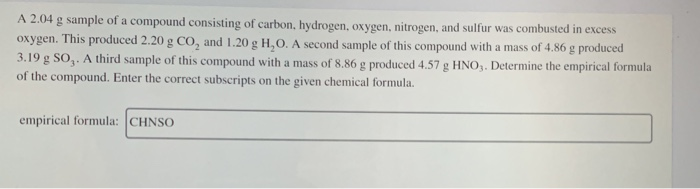f a compound consisting of carbon, hydrogen, oxygen, nitrogen, and sulfur was combusted in excess oxygen. This produced 2.20 g Co2 and 1.20 g H,O. A second sample of this compound with a mass of 4.86 g produced 3.19 g SO,. A third sample of this compound with a mass of 8.86 g produced 4.57 g HNO,. Determine the empirical formula of the compound. Enter the correct subscripts on the given chemical formula. empirical formula: CHNSO f a compound consisting...

• 002 14. When 10.0 g of phosphorous reacted with oxygen 100 g of phosphorous reacted with...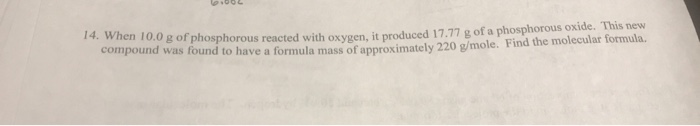002 14. When 10.0 g of phosphorous reacted with oxygen 100 g of phosphorous reacted with oxygen, it produced 17.77 g of a phosphorous oxide. This new compound was found to have a formula mass of approximately 220 g/mole. Find the molecular formula,

• 7. Solid sodium oxide is synthesized from it elements in a reaction that is 45.6% efficient....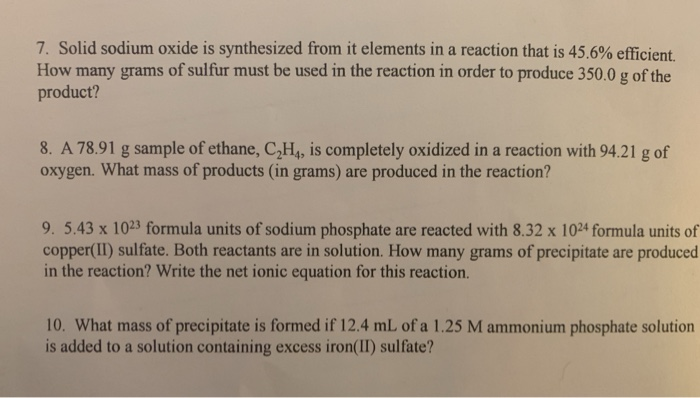7. Solid sodium oxide is synthesized from it elements in a reaction that is 45.6% efficient. How many grams of sulfur must be used in the reaction in order to produce 350.0 g of the product? 8. A 78.91 g sample of ethane, C,H, is completely oxidized in a reaction with 94.21 g of oxygen. What mass of products (in grams) are produced in the reaction? 9. 5.43 x 1023 formula units of sodium phosphate are reacted with 8.32 x...

• 7. A 0.250-g sample of calcium metal is heated in a 38.500-g crucible to form calcium...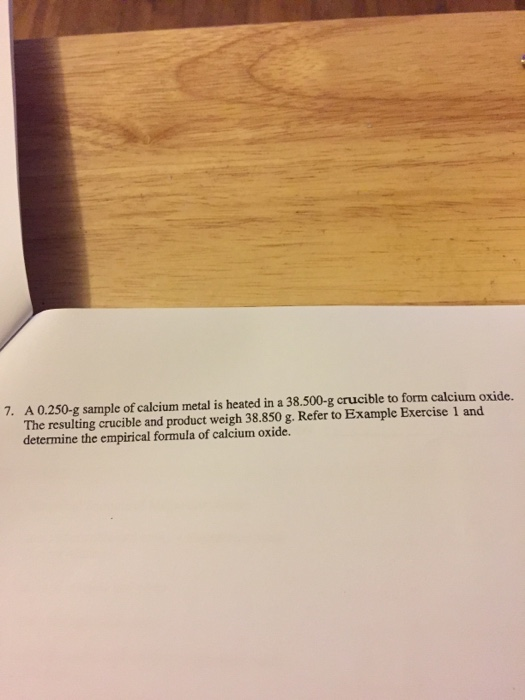7. A 0.250-g sample of calcium metal is heated in a 38.500-g crucible to form calcium oxide. The resulting crucible and product weigh 38.850 g. Refer to Example Exercise 1 and determine the empirical formula of calcium oxide. DATE DATA TABLE A Empirical Formula of Magnesium Oxide mass of crucible and cover + magnesium metal (before heating) mass of crucible and cover mass of magnesium metal mass of crucible and cover + magnesium oxide (after heating ) 0000 mass of...

• A 2.75 g sample of a compound consisting of carbon, hydrogen, oxygen, nitrogen, and sulfur was combusted in excess oxyg...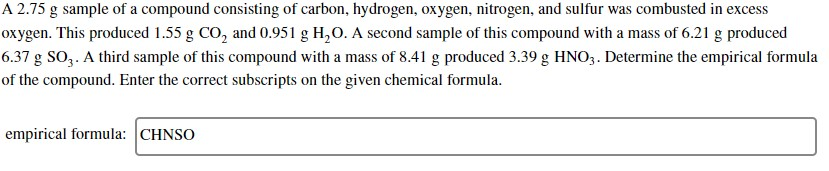A 2.75 g sample of a compound consisting of carbon, hydrogen, oxygen, nitrogen, and sulfur was combusted in excess oxygen. This produced 1.55 g CO, and 0.951 g H,O. A second sample of this compound with a mass of 6.21 g produced 6.37 g SOZ. A third sample of this compound with a mass of 8.41 g produced 3.39 g HNO3. Determine the empirical formula of the compound. Enter the correct subscripts on the given chemical formula. empirical formula: CHNSO

• Calculate the mass of water produced when 7.44 g of butane reacts with excess oxygen. Express...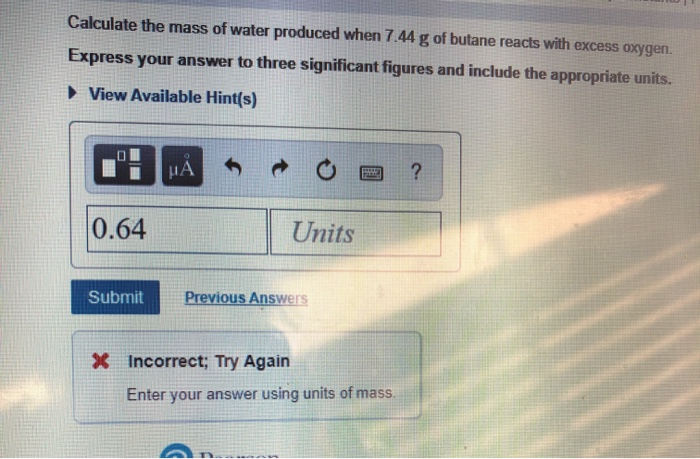Calculate the mass of water produced when 7.44 g of butane reacts with excess oxygen. Express your answer to three significant figures and include the appropriate units. View Available Hint(s) 0.64 Units Submit Previous Answers * Incorrect; Try Again Enter your answer using units of mass. Part Review Constants Periodic Table Calculate the mass of butane needed to produce 61.3 g of carbon dioxide. Express your answer to three significant figures and include the appropriate units. ► View Available Hint(s)...

• Question When 10.00 g of magneslum are burned, 16.58 g of magnesium oxide are produced. What...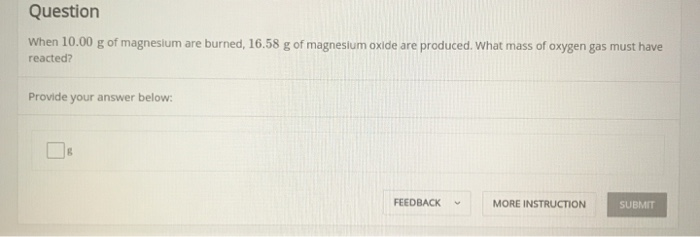Question When 10.00 g of magneslum are burned, 16.58 g of magnesium oxide are produced. What mass of oxygen gas must have reacted? Provide your answer below FEEDBACKMORE INSTRUCTION SUBMIT

• compound is found to contain 63.65 % nitrogen and 36.35 % oxygen by mass. the empirical...

compound is found to contain 63.65 % nitrogen and 36.35 % oxygen by mass. the empirical formula is? the molar mass is 44.02 g/mol. what is the molecular formula? A compound is found to contain 6.360 % silicon , 36.18 % bromine , and 57.46 % iodine by mass. the empirical formula is? the molar mass is 441.7. what is the molecular formula? A compound is found to contain 64.80 % carbon , 13.62 % hydrogen , and 21.58 %...